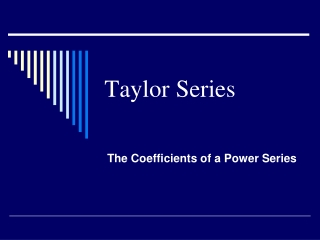DownloadDownload PresentationTaylor Series

# Taylor Series

Télécharger la présentation## Taylor Series

- - - - - - - - - - - - - - - - - - - - - - - - - - - E N D - - - - - - - - - - - - - - - - - - - - - - - - - - -
##### Presentation Transcript

1. Taylor Series The Coefficients of a Power Series

2. Coefficients of a Power Series Suppose that we have function f given by a power series What can we say about the relationship between f and the coefficients a0, a1, a2, a3, a4, a5, . . ? Answer: Quite a bit, and the reasoning should look somewhat familiar to you. Here’s how it goes . . .

3. Furthermore, Theorem 13 (pg. 591 in OZ), says that Thus If Then Is this beginning to look familiar? It should remind you of the process by which we computed the coefficients of the Taylor polynomial approximations

4. Continuing to take derivatives and evaluate at x0, we have . . .

5. In general, we have: which tells us that In other words, if a function f is given by a power series that is centered at x0, that power series must be the Taylor series for f based at x0. If, we have Then

6. It is easy to see that Taylor series are just a special kind of power series. Our discovery tells us that they are really the only kind of power series there is. To reiterate: If a function f is given by a power series, that power series must be the Taylor Series for f at the same base point. • Notice what this does not say. • It does not say that every function is given by its Taylor Series. • It does not even say that every function that has a Taylor series is given by its Taylor Series.

7. For a Function f, Some Questions Arise • If f has a Taylor Series, does the series converge? Answer: Often, but not always, and certainly not always on the whole domain of the function. • Consider the familiar case of What is the Taylor Series for this function? What can we say about its convergence?

8. Next Question . . . • If the Taylor Series for f converges, is it equal to the f on its interval of convergence? Answer: Often, but not always. • Consider the absolute value function We know that we cannot expand it in a Taylor series about x=0. (Why?) But f (x) = | x | has derivatives of all orders at all other points. What if we consider a Taylor series expansion about x = 1?

9. Taylor Series for f (x)= |x| based at x = 1. What about the derivatives of f at x = 1? What do we get for the Taylor series expansion at x = 1? The Taylor Series expansion for f (x) = | x | converges on the entire real line, but is equal to f only on the interval [0,∞)!

10. Things can get reallyweird • Facts: • f is continuous and has derivatives of all orders at x = 0. • f (n)(0)=0 for all n. What does all this tell us about the Maclaurin Series for f ? The Maclaurin Series for f converges everywhere, but is equal to f only at x = 0!

11. So where does this leave us? • To Summarize: • Even if we can compute the Taylor Series for a function, • the Taylor Series does not always converge. • If the Taylor Series converges, the Taylor Series is not necessarily equal to the function, even on its interval of convergence. We know how to determine whether (and where) the Taylor series converges---Ratio test! But how do we know if the Taylor Series of the function is equal to the function on the interval where it converges?

12. What does this tell us? • Pointing out that a Taylor series for f “might converge at all x but perhaps to a limit other than f,” Ostebee and Zorn assure us that • “Taylor’s theorem guarantees that this unfortunate event seldom occurs.” • Pinning that down is the subject of our next lesson.